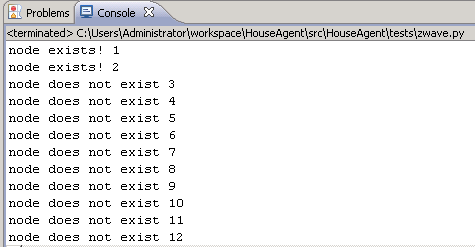# Z-wave and python node discovery, more bitwise trickery

This post continues upon the last blog post, in the last post we looked at some z-wave protocol basics.
At the same time we had a look at a bitwise operation (XOR), in this post some more bitwise tricks will be used to do z-wave node discovery.
Node discovery is essential to get information about the members of a z-wave network.
The serial port sniff in the previous post, showed the FUNC_ID_SERIAL_API_GET_INIT_DATA command. Let’s dive into this packet…
Here’s the packet response again:
#06#01#25#01#02#05#00#1D#03#00#00#00#00#00#00#00#00#
00#00#00#00#00#00#00#00#00#00#00#00#00#00#00#00#00#00#00#00#03#01#C0

The first 4 bytes are not relevant for this post, I have to look into them later on. The fun starts at byte “02”, this byte represents the function response code (in this case FUNC_ID_SERIAL_API_GET_INIT_DATA). The byte following (05) is the z-wave protocol version, in this case v5. Next byte is “00” which is the controller type (primary or secondary)
“1D” indicates the amount of node bytes. 1D is 29 decimal.
Z-wave uses these node bytes to indicate whether or not a node is in use within the network (the bytes are bit masked). For example the first node byte value 03, is as follows in binary:
0000 0011

This means node 1 and 2 are in use, another example:

0000 1011

This would indicate node 4, 2 and 1 being used. So, how do we figure this out from code? This is where bitwise AND comes into play. A bitwise AND takes two binary representations of equal length and performs the logical AND operation on each pair of corresponding bits. In each pair, the result is 1 if the first bit is 1 AND the second bit is 1. Otherwise, the result is 0.
We can use this from our python code in the following matter:

```    length = ord(response) - 1
index = 1

for i in range(0, length):
buffer_index = response[8+i]
num = 1
j = 0

while j <= 7:
if (ord(buffer_index) & num > 0):
print "node exists!", index
else:
print "node does not exist", index

index = index+1

if j < 7:
num = num * 2

j = j+1
```

And does it work?Looks fine!

Maarten

Hi, I am Maarten and I am the owner of this weblog. I post on various IT subjects that matter in my life as an IT professional.